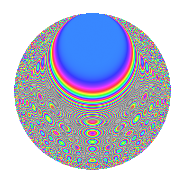# Properties

 Label 1890.2.bwLevel 1890 Weight 2 Character orbit bw Rep. character $$\chi_{1890}(557,\cdot)$$ Character field $$\Q(\zeta_{12})$$ Dimension 192 Sturm bound 864

# Related objects

## Defining parameters

 Level: $$N$$ = $$1890 = 2 \cdot 3^{3} \cdot 5 \cdot 7$$ Weight: $$k$$ = $$2$$ Character orbit: $$[\chi]$$ = 1890.bw (of order $$12$$ and degree $$4$$) Character conductor: $$\operatorname{cond}(\chi)$$ = $$315$$ Character field: $$\Q(\zeta_{12})$$ Sturm bound: $$864$$

## Dimensions

The following table gives the dimensions of various subspaces of $$M_{2}(1890, [\chi])$$.

Total New Old
Modular forms 1824 192 1632
Cusp forms 1632 192 1440
Eisenstein series 192 0 192

## Trace form

 $$192q$$ $$\mathstrut +\mathstrut O(q^{10})$$ $$192q$$ $$\mathstrut +\mathstrut 96q^{16}$$ $$\mathstrut -\mathstrut 36q^{17}$$ $$\mathstrut -\mathstrut 12q^{41}$$ $$\mathstrut -\mathstrut 12q^{46}$$ $$\mathstrut +\mathstrut 48q^{50}$$ $$\mathstrut +\mathstrut 24q^{58}$$ $$\mathstrut +\mathstrut 12q^{61}$$ $$\mathstrut -\mathstrut 24q^{65}$$ $$\mathstrut +\mathstrut 48q^{77}$$ $$\mathstrut +\mathstrut 24q^{92}$$ $$\mathstrut +\mathstrut O(q^{100})$$

## Decomposition of $$S_{2}^{\mathrm{new}}(1890, [\chi])$$ into irreducible Hecke orbits

The newforms in this space have not yet been added to the LMFDB.

## Decomposition of $$S_{2}^{\mathrm{old}}(1890, [\chi])$$ into lower level spaces

$$S_{2}^{\mathrm{old}}(1890, [\chi]) \cong$$ $$S_{2}^{\mathrm{new}}(315, [\chi])$$$$^{\oplus 4}$$$$\oplus$$$$S_{2}^{\mathrm{new}}(630, [\chi])$$$$^{\oplus 2}$$$$\oplus$$$$S_{2}^{\mathrm{new}}(945, [\chi])$$$$^{\oplus 2}$$